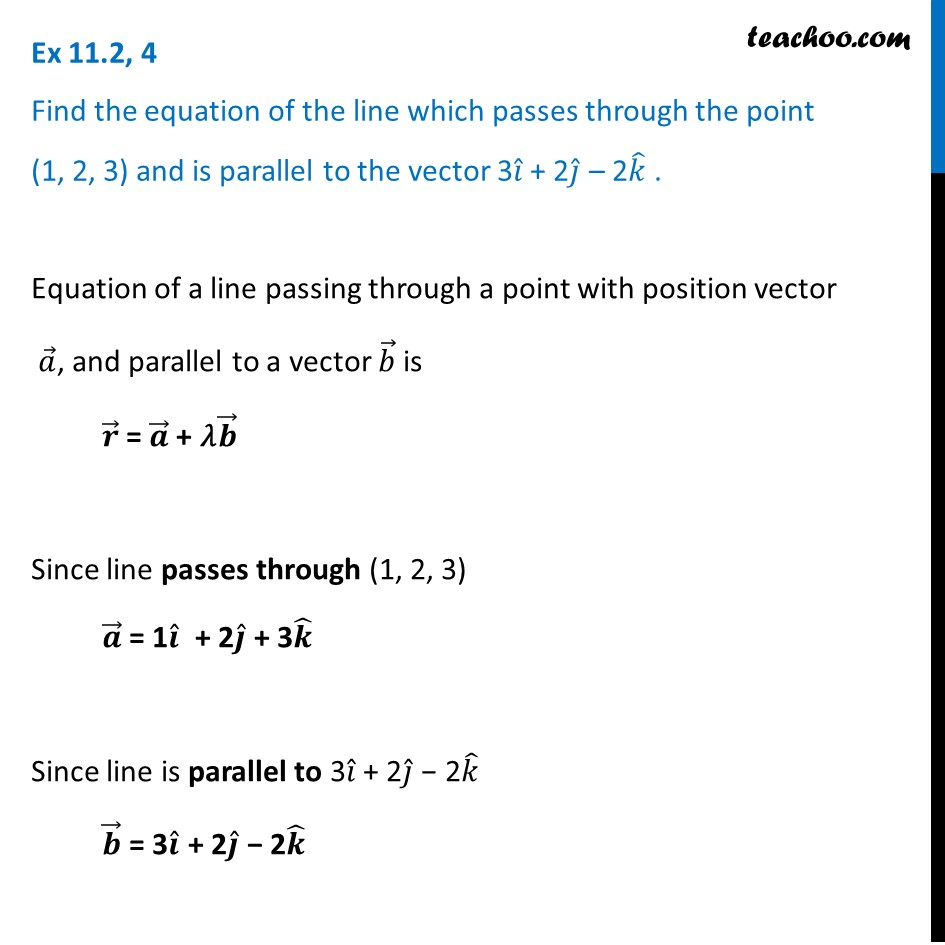Ex 11.2

Chapter 11 Class 12 Three Dimensional Geometry
Serial order wiseLearn in your speed, with individual attention - Teachoo Maths 1-on-1 Class

### Transcript

Ex 11.2, 4 Find the equation of the line which passes through the point (1, 2, 3) and is parallel to the vector 3𝑖 ̂ + 2𝑗 ̂ – 2𝑘 ̂ . Equation of a line passing through a point with position vector 𝑎 ⃗, and parallel to a vector 𝑏 ⃗ is 𝒓 ⃗ = 𝒂 ⃗ + 𝜆𝒃 ⃗ Since line passes through (1, 2, 3) 𝒂 ⃗ = 1𝒊 ̂ + 2𝒋 ̂ + 3𝒌 ̂ Since line is parallel to 3𝑖 ̂ + 2𝑗 ̂ − 2𝑘 ̂ 𝒃 ⃗ = 3𝒊 ̂ + 2𝒋 ̂ − 2𝒌 ̂ Equation of line 𝑟 ⃗ = 𝑎 ⃗ + 𝜆𝑏 ⃗ 𝒓 ⃗ = (𝒊 ̂ + 2𝒋 ̂ + 3𝒌 ̂) + 𝜆 (3𝒊 ̂ + 2𝒋 ̂ − 2𝒌 ̂)